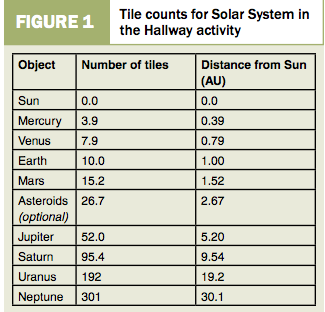Hallway Solar System:

Your team is going to go into the hallway and create a hallway solar system.  You need to read the Hallway Solar System website and follow the directions there.

Before you begin, answer the following questions:

1. Can you state the order of the planets from nearest to the Sun to farthest away?

2. Do you think the inter-planet distances (distances between neighboring planets) are uniform?

3. If not, is there a pattern to the intervals or are the differences random?

4. Suppose we say the Sun is at one end of the hall and we scale the solar system so the Earth is 10 tiles from the Sun. Do you think all of the solar system will fit in the hallway? If so, where will the farthest planet from the Sun be located?

What to do:

1. Make a name tag for each of the planets and the asteroid belt.

2. Gather a set of balls to represent the planets and the Sun.

3. Go into the hallway and QUIETLY place the objects the correct distances from the “sun”.  Use the chart in Figure 1 to see how far apart to place the “planets”.

Use the floor tiles.1. By sight, what is the closest object-to-object distance? Record the names of the objects and your estimate of the distance in number of tiles.

2. By sight, what is the greatest distance between two adjacent objects? Record the names of the planets (or objects) and the distance between them (in number of tiles).

3.  Record the distance (in number of tiles) from the Sun to Mercury.

4.  How far (in tiles) is it from the Sun to Earth?

5.  When you are at Earth, record how big the Sun appears using your ruler.

6.  How far (in tiles) is it from Earth to Jupiter?

7.  How long does it take to get from the Sun to Neptune using the heel-to-toe method?

8.  How far is Uranus from Neptune (in tiles)?

9.  When you are at Neptune, record how big the Sun appears.   The size of the “Sun,”  can be measured at each position (Earth, Jupiter, and Neptune) by holding a ruler at arm’s length to determine the apparent diameter of the Sun picture or the length of the sign.  Write down how many centimeters in diameter the Sun appears.

From Earth=                             From Mercury=                            From Jupiter=

From Saturn=                           From Neptune=

Extra Credit Extension Activities:

1. Given the distance data for Ceres, Pluto, and Eris, where would the three dwarf planets be on our scale? Would all of them fit in our hallway? [Ceres is 415 million km from the Sun (The StarChild Team, Ceres)]. Pluto is 5.87 billion km from the Sun (Spinrad 2004). Eris is about 10 billion km from the Sun (The StarChild Team, Eris). 1 au= 150 million km. Earth is 150,000,000 from the Sun.  Use these numbers to calculate the number of tiles for this question.

2. Students may wish to use the internet to gather information that would allow them to calculate how long it would take for a spaceship from Earth to reach Mars (the first step people consider with respect to exploring the solar system). Time to reach more distant planets can also be calculated, if desired.

3. What has to be considered in planning for humans to travel to and to explore on Mars? Think about both the trip to Mars, exploration phase, and the return trip to Earth.